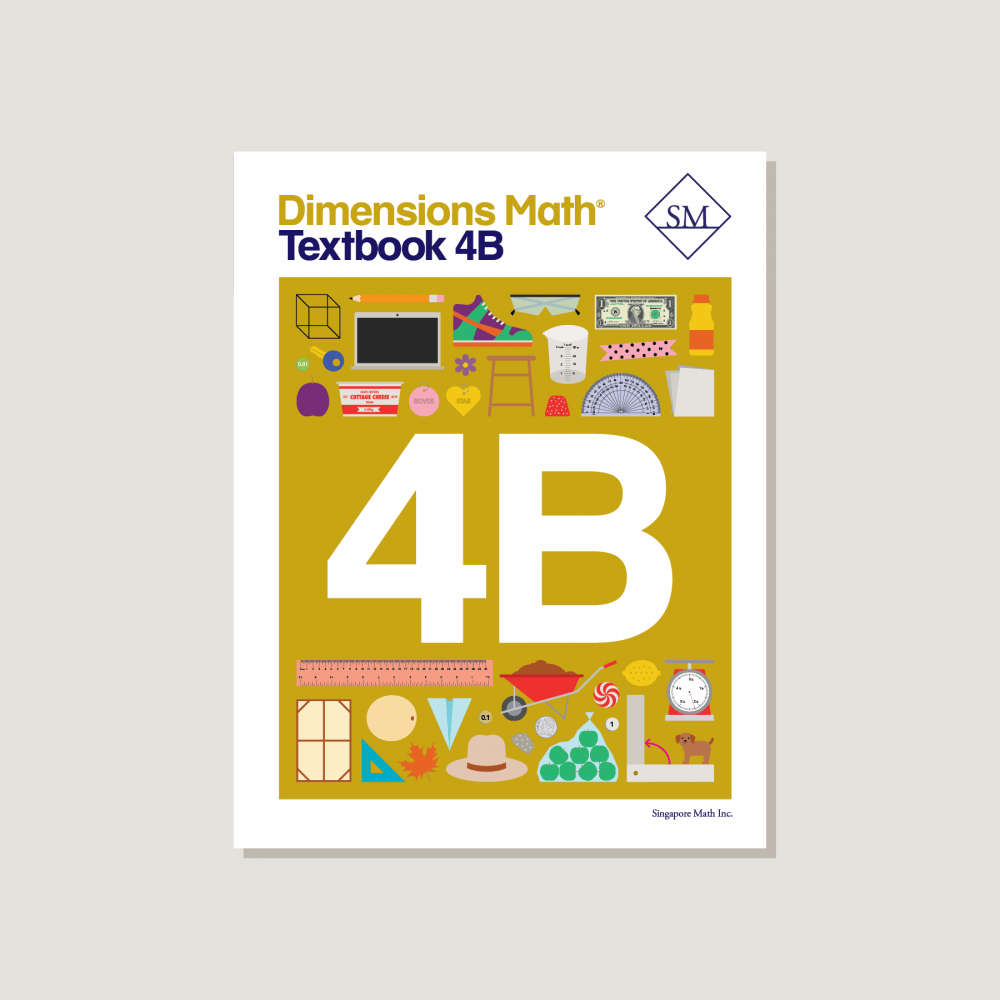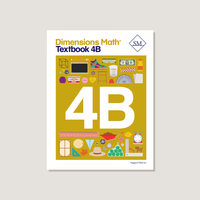# Dimensions Math Textbook 4B

\$12.80

Condition New

Textbooks introduce concepts through logical sequencing. Lessons encourage discussion and help students reach fluency through engaging activities and strategies. Corresponding exercises in workbooks help students consolidate and practice new knowledge. Features & Components:
• Chapter Opener: Each chapter begins with an engaging scenario that stimulates student curiosity in new concepts. This scenario also provides teachers an opportunity to review skills.
• Think: With guidance from teachers, students solve a problem using a variety of methods.
• Learn: One or more solutions to the problem in Think are presented, along with definitions and other information to consolidate the concepts introduced in Think.
• Do: A variety of practice problems allow teachers to lead discussion or encourage independent mastery. These activities solidify and deepen student understanding of the concepts.
• Exercise: A pencil icon at the end of the lesson links to additional practice problems in the corresponding workbook.
• Practice: Periodic practice provides teachers with opportunities for consolidation, remediation, and assessment.
• Review: Two cumulative reviews provide ongoing practice of concepts and skills.
Note: Two textbooks (A and B) for each grade correspond to the two halves of the school year. Answer key not included. Soft cover.
SKU: DMT4B

ISBN: 9781947226111

Pagecount: 248

Dimensions: 8.5 x 11 x 0.75 in

Weight: 1.5 lbs

Binding: Standard

Color: Color

Cover: Soft

Perforated: No

Sample Pages

Chapter 10: Measurement
Lesson 1: Metric Units of Measurement
Lesson 2: Customary Units of Length
Lesson 3: Customary Units of Weight
Lesson 4: Customary Units of Capacity
Lesson 5: Units of Time
Lesson 6: Practice A
Lesson 7: Fractions and Measurement — Part 1
Lesson 8: Fractions and Measurement — Part 2
Lesson 9: Practice B

Chapter 11: Area and Perimeter
Lesson 1: Area of Rectangles — Part 1
Lesson 2: Area of Rectangles — Part 2
Lesson 3: Area of Composite Figures
Lesson 4: Perimeter — Part 1
Lesson 5: Perimeter — Part 2
Lesson 6: Practice

Chapter 12: Decimals
Lesson 1: Tenths — Part 1
Lesson 2: Tenths — Part 2
Lesson 3: Hundredths — Part 1
Lesson 4: Hundredths — Part 2
Lesson 5: Expressing Decimals as Fractions in Simplest Form
Lesson 6: Expressing Fractions as Decimals
Lesson 7: Practice A
Lesson 8: Comparing and Ordering Decimals
Lesson 9: Rounding Decimals
Lesson 10: Practice B

Chapter 13: Addition and Subtraction of Decimals
Lesson 1: Adding and Subtracting Tenths
Lesson 2: Adding Tenths with Regrouping
Lesson 3: Subtracting Tenths with Regrouping
Lesson 4: Practice A
Lesson 6: Subtracting from 1 and 0.1
Lesson 7: Subtracting Hundredths
Lesson 8: Money, Decimals, and Fractions
Lesson 9: Practice B
Review 3

Chapter 14: Multiplication and Division of Decimals
Lesson 1: Multiplying Tenths and Hundredths
Lesson 2: Multiplying Decimals by a Whole Number — Part 1
Lesson 3: Multiplying Decimals by a Whole Number — Part 2
Lesson 4: Practice A
Lesson 5: Dividing Tenths and Hundredths
Lesson 6: Dividing Decimals by a Whole Number — Part 1
Lesson 7: Dividing Decimals by a Whole Number — Part 2
Lesson 8: Dividing Decimals by a Whole Number — Part 3
Lesson 9: Practice B

Chapter 15: Angles
Lesson 1: The Size of Angles
Lesson 2: Measuring Angles
Lesson 3: Drawing Angles
Lesson 4: Adding and Subtracting Angles
Lesson 5: Reflex Angles
Lesson 6: Practice

Chapter 16: Lines and Shapes
Lesson 1: Perpendicular Lines
Lesson 2: Parallel Lines
Lesson 3: Drawing Perpendicular and Parallel Lines
Lesson 5: Lines of Symmetry
Lesson 6: Symmetrical Figures and Patterns
Lesson 7: Practice

Chapter 17: Properties of Cuboids
Lesson 1: Cuboids
Lesson 2: Nets of Cuboids
Lesson 3: Faces and Edges of Cuboids
Lesson 4: Practice
Review 4
Review 5

A & B Books: Our programs divide the school year into two semesters. “A” level books are for the first half of the school year. “B” level books are for the second half of the school year. You need both “A” and “B” material for a complete school year.

Required Components: Textbooks, Workbooks, and Guides (either Home Instructor’s Guides or Teacher’s Guides) are all necessary components. These three elements each serve a unique function and work together to build math mastery.

Printouts: Dimensions Math PK-5 Resources

Recommended Manipulatives: Dimensions Math Grades PK-5 Recommended Manipulatives

Answer Key: Located in corresponding Teacher’s Guide

Dimensions Math At Home™ Videos

Invite a professional Singapore math teacher into your home classroom. This subscription of pre-recorded lessons covers all Textbook and Workbook material for an entire school year and can be done at your own pace.

Manipulatives

Manipulatives help students visualize and represent math concepts. Stock up on suggested items to deepen engagement during math activities and lessons.

Supplementary Math

Supplementary math helps students of all abilities: struggling, advanced, or on level. Find just the right addition to round out your core program.